## teaching and educationJOURNAL OFSYNCHROTRONRADIATION
ISSN: 1600-5775
Volume 26| Part 6| November 2019| Pages 2094-2096

## An enlightening procedure to explain the extreme power of synchrotron radiation

aFaculté des Sciences de Base, Ecole Polytechnique Fédérale de Lausanne (EPFL), CH 1015 Lausanne, Switzerland
*Correspondence e-mail: giorgio.margaritondo@epfl.ch

(Received 20 March 2019; accepted 5 August 2019; online 10 October 2019)

A simple approach exploits quantum properties to justify the dependence on γ4 of the total synchrotron emitted power. It also clarifies some apparent puzzles and brings to light the underlying, multiple relativistic phenomena.

The relativistic arguments that explain the key properties of synchrotron radiation are sometimes tricky and potentially confusing for students (Margaritondo & Rafelski, 2017). One effective way to fight against the confusion is to use quantum properties (Margaritondo, 1995, 2019). We present here a specific example: a derivation of the total emitted power of a synchrotron source; and a comparison of the result with the Lorentz transformations for the energy of the radiation and its intensity.

The key point is of course that the total emitted power measured in the laboratory is proportional to γ4 (Margaritondo, 1988; Mobilio et al., 2015), where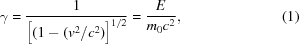v is the longitudinal speed of the emitting electron, E is the energy and m0 is the rest mass. The γ4-dependence is one of the most fundamental properties of synchrotron radiation (Margaritondo, 1988; Mobilio et al., 2015), notably contributing to the extreme brightness and causing massive hadrons to emit much less than leptons.

However, the γ4 factor may be somewhat puzzling: it is sometimes difficult for students to reconcile it with the Lorentz transformation of the wave energy from the electron reference frame to the laboratory frame (Rafelski, 2017; Steane, 2011; Einstein, 1905b):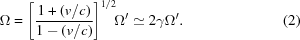Likewise, it might seem at odds with the transformation of the intensity (Einstein, 1905b)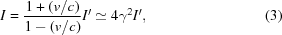which, by the way, led Einstein as early as in 1905 to implicitly anticipate the high brightness of synchrotron radiation (Margaritondo & Rafelski, 2017; Einstein, 1905a).

Note that the total power emitted by an accelerated electron is a relativistic invariant. In fact, in the electron reference frame it is equal to the decrease per unit time of the electron energy m0c2. In another inertial frame the electron energy becomes γm0c2 and relativistic dilation changes the time also by a factor of γ, leaving the emitted power unchanged. This, however, does not help justifying its γ4-dependence.

The standard way to derive the γ4-dependence (Margaritondo, 1988) is based on the Lorentz transformations of time, coordinates and ultimately accelerations, and is quite straightforward. But it does not clarify the above apparent discrepancies. And, as we shall realize, it does not bring to light all the relativistic phenomena that underlie the γ4-dependence.

Quantum properties offer an alternate approach, which besides being simple also helps us to solve the above puzzles. Consider a synchrotron radiation source and suppose that its emission is quantized (Einstein, 1905a), i.e. when detected it behaves like a collection of photons. Assume that a photon counter reveals the radiation that reaches its small capture area S, perpendicular to the (narrow) forward synchrotron beam (note that the transverse area S is Lorentz-invariant).

Suppose that the detector is synchronized with the electron bunches so that it counts only photons from electrons emitting at a distance D from S during a short time period δt. Furthermore, assume that the detector includes spectral filtering and only counts photons of energy hν, corresponding to the maximum of the emission spectrum. Defining P(hν) as the emitted power at that photon energy measured in the laboratory reference frame, the photon counts during δt can be written as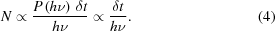Going now to the electron frame, the synchrotron emission is no longer confined to a narrow beam but occurs over a broad angular range – and the detector surface S only catches a portion. Assume, oversimplifying a bit, that the emission is isotropic: the portion that reaches S would be S/(4πD2), where D′ is the electron–detector distance in the electron frame. Compared with D, D′ is Lorentz contracted (Rafelski, 2017; Steane, 2011),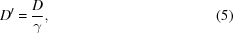so the photon counts in the electron frame can be written as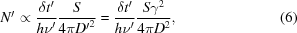where δt′ and hν′ are the detection time and the detected photon energy measured in the electron frame. The more correct `cosine square' (Larmor) law for the angular distribution of the emission in the electron reference frame would require calculation of a surface integral,1 but would not change the essential point of equation (6),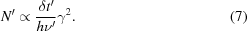The photon counts given by equations (4)and (7)must of course coincide; after all, they could be read in a numerical display and the readings would be the same in both reference frames. Thus,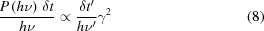and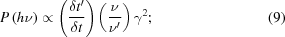the two factors in parentheses in equation (9)are equal to γ (reciprocal time dilation) and, approximately, to 2γ (Doppler effect; Rafelski, 2017; Steane, 2011). Thus, we have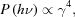which also explains the γ4-dependence of the total emitted power.

What do we learn from this alternate approach? First, that the γ4-dependence of the emitted power cannot be derived from the transformation of the radiation energy alone, nor only from that of the intensity, because it is caused by a more complex combination of different relativistic phenomena, i.e. Lorentz contraction, time transformation and the Doppler effect.

By correctly treating these effects, one can realize that the above discrepancies are apparent, and in each case the treatment can be simplified by shortcuts offered by quantum properties.

For example, the radiation energy corresponding to photons of energy hν can be written as a photon count multiplied by hν. Thus, since photon counts are invariant, the radiation energy and the frequency must transform in the same way. This explains why the factor of equation (2)is the same as that of the Doppler effect (Rafelski, 2017).

Regarding the intensity, note that it is equal to the radiation energy density multiplied by the invariant c, so it must transform like the energy density. Consider now a volume defined by S and by a length equal to n wavelengths λ = c/ν (in the laboratory frame), which contains A photons of energy hν. The energy density corresponding to such photons is Ahν/Sn(c/ν) = [Ah/(Snc)]ν2 in the laboratory frame and [Ah/(Snc)]ν2 in the electron frame. Thus, since the term Ah/Snc only includes invariants, the energy density and therefore the intensity must transform in the same way as ν2 – justifying equation (3), whose factor is indeed the square of the Doppler factor.

Finally, to correctly relate the intensity to the total power one must not make the mistake of assuming that they transform in the same way because S is invariant. In fact, as explained above, the solid angle captured by S is not invariant due to the Lorentz contraction. And this, as we have seen, introduces the additional factor proportional to γ2 that transforms equation (3)into the γ4-dependence of the emitted power.

This is, in general terms, a specific example of a more systematic aspect (Margaritondo & Rafelski, 2017; Margaritondo, 1988); many important properties of synchrotron radiation are the result of not one but several combined relativistic effects. Only by taking into account all these effects can one avoid apparent contradictions and serious mistakes.

### Footnotes

1Specifically, the integral of 2πD2sinθ (where θ is the angle with respect to the longitudinal direction), used to calculate the spherical surface area including S, has to be replaced by the integral of 2πD2sinθcos2θ, obtaining 4πD2/3 instead of 4πD2.

### Funding information

This work was supported by the Ecole Polytechnique Fédérale de Lausanne (EPFL) (award to GM).

### References

© International Union of Crystallography. Prior permission is not required to reproduce short quotations, tables and figures from this article, provided the original authors and source are cited. For more information, click here.JOURNAL OFSYNCHROTRONRADIATION
ISSN: 1600-5775
Volume 26| Part 6| November 2019| Pages 2094-2096
Follow J. Synchrotron Rad.